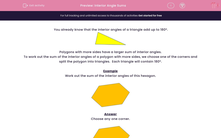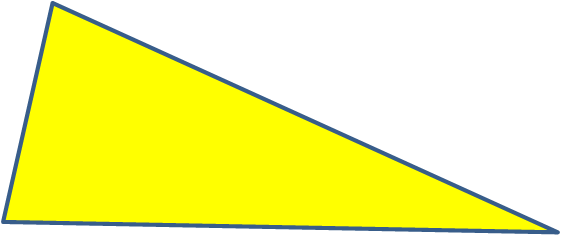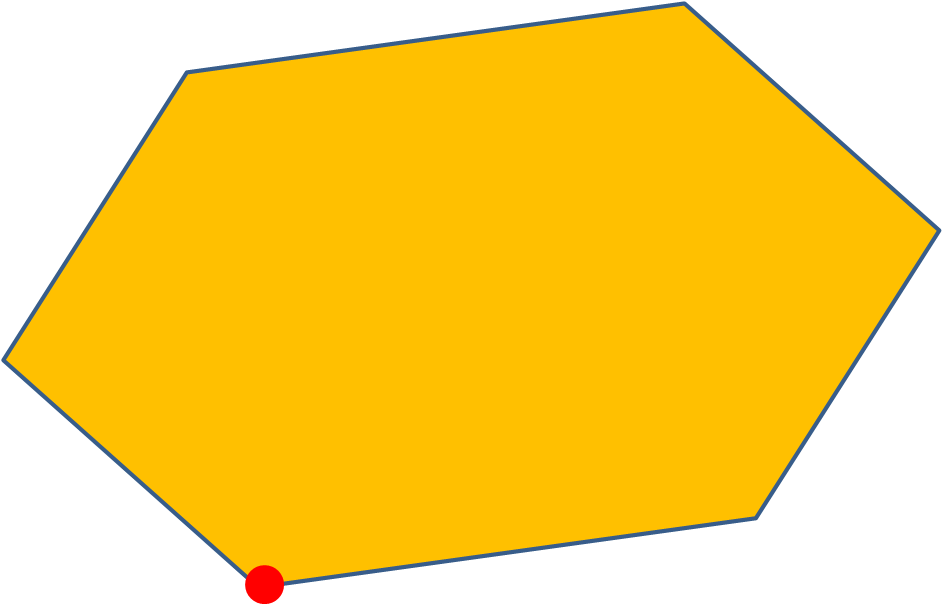# Interior Angle Sums

In this worksheet, students calculate the sum of the interior angles of the given polygons.Key stage:  KS 2

Curriculum topic:   Maths and Numerical Reasoning

Curriculum subtopic:   2D Shapes: Circles, Angles and Bearings

Difficulty level:#### Worksheet Overview

You already know that the interior angles of a triangle add up to 180º.Polygons with more sides have a larger sum of interior angles.

To work out the sum of the interior angles of a polygon with more sides, we choose one of the corners and split the polygon into triangles.  Each triangle will contain 180°.

Example

Work out the sum of the interior angles of this hexagon.Choose any one corner.Draw diagonals from the chosen corner to form triangles.There are 4 triangles inside the hexagon.

4 x 180° = 720°

The sum of the interior angles of a hexagon is 720°.

General Rule:

When you have a shape with n sides, the number of triangles it can be split into is n-2

Once you have the number of triangles, we can simply multiply that by 180° to find the internal angles.

For example: A 5 sided shape can be split into 3 triangles, if you then multiply 3 by 180 you get that there are 540° in a 5 sided shape.

### What is EdPlace?

We're your National Curriculum aligned online education content provider helping each child succeed in English, maths and science from year 1 to GCSE. With an EdPlace account you’ll be able to track and measure progress, helping each child achieve their best. We build confidence and attainment by personalising each child’s learning at a level that suits them.

Get started